Speakers, Talks and Abstracts

Location: Ruppert Blauw lecture room in the Educatorium building in the Utrecht Science Park (de Uithof). See map.Anton Alekseev
Geneva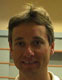Philip Boalch
ENS Paris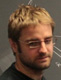Damien Calaque
ETH and LyonGil Cavalcanti
Utrecht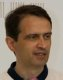Giovani Felder
ETH-ZürichMarco Gualtieri
University of TorontoVictor Guillemin
MITSergei Gukov
CaltechNigel Higson
Penn StateNigel Hitchin
Oxford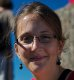Göttingen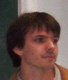Camille Laurent-Gengoux
MetzDavid Li-Bland
TorontoJiang-Hua Lu
Hong Kong

David Martinez-Torres
IST LisbonRajan Mehta
Penn StateYoshihiko Mitsumatsu
TokyoHessel Posthuma
UvAETH-ZürichFlorian Schaetz
Utrecht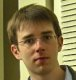Mathieu Stienon
Penn StateAlan Weinstein
UC Berkeley

Thomas Willwacher
Harvard

Titles and Abstracts

Opening Session - video

Anton Alekseev (Geneva) - video

Title: Poisson-Lie groups and tropical correspondence principle

Abstract: A Poisson-Lie group is a Lie group $G$ carrying a Poisson bracket $\pi$ which makes the group multiplication into a Poisson map. For $t$ a real number, $\pi_t = t \pi$ defines a one-parameter family of Poisson structures on $G$. For $K$ is a compact Lie group, the Poisson structures $\pi_t$ on $K^*$ are all isomorphic to each other (for $t$ non-vanishing) by the Ginzburg-Weinstein Theorem. The linearization technique shows that many other features of $K^*$ equipped with the Poisson bracket $\pi_t$ are independent of $t$.

The tropical correspondence principle is a tool in algebraic geometry which is based on the following observation: for a certain type of algebraic equations, one can introduce a parametrization of variables using a real parameter $t$. The $t \to \infty$ limit makes sense, and leads to a combinatorial problem which is usually more tractable than the algebraic problem that one started with.

In the talk, we show how to pass to the limit $t \to \infty$ in the case of the Poisson-Lie group $U(n)^*$. In particular, we obtain a combinatorial version of the Horn problem on the eigenvalues of triples of Hermitian matrices which add up to zero. In this case, we use Liouville volumes and the Gelfand-Zeitlin integrable system to establish the correspondence between tropical ($t= \infty$) and non-tropical solutions.

The talk is based on joint works with M. Podkopaeva and A. Szenes.

Philip Boalch (ENS Paris) - video & slides

Title: Poisson geometry of moduli spaces of meromorphic connections on curves and their Stokes data

Abstract: For G=GL_n(C) the space of flat G connections on a punctured complex algebraic curve is the same as the space of holomorphic connections, and in turn, via Deligne's Riemann-Hilbert correspondence, it is the same as the space of regular singular connections on algebraic vector bundles. Now, on a curve, a connection is regular singular if and only if it is the restriction of a meromorphic connection on the compact curve which only has simple poles at the marked points. In this talk I'll describe how one may relax this regularity assumption and explain how the quasi-Hamiltonian approach may be extended to this, irregular, context. In particular a new operation "fission" arises, complementary to fusion. Fission enables us to do induction with respect to the pole order, whereas fusion enables induction with respect to the genus and number of poles. This yields a rich class of holomorphic Poisson varieties, the "wild character varieties". I'll also describe the natural generalization of the well-known braid and mapping class group actions (the simplest case of which is known to account for the quantum Weyl group actions).

Damien Calaque (ETH and Lyon) - video

Title: Classical TFTs and symplectic structures on mapping spaces with boundary conditions

Abstract: In this talk I will present an extension of a recent result of Pantev-To 'en-Vaqui'e-Vezzosi on the construction of shifted symplectic structures on derived mapping stacks (which can be viewed as an approach to the AKSZ construction that avoids problems involving infinite dimension). The main new ingredient compare to PTVV is the presence of boundary conditions, which are necessary if one wants to understand the work of Cattaneo-Felder on the Poisson sigma-model. I will also explain how this is related to the project of constructing various extended Topological Field Theories with values in an appropriate category of Lagrangian correspondences (inspired by the recent work of Cattaneo-Mnev-Reshetikhin).

Gil Cavalcanti (Utrecht) - video & slides

Title: SKT geometry

Abstract: In classical terms, an SKT structure is a Hermitian structure for which the Hermitian 2-form is closed with respect to the second order operator dd^c. These structures arise naturally in the study of sigma models with (2,0) or (2,1)-supersymmetries, much like generalized Kahler structures arise in the (2,2)-supersymmetric sigma model. While the introduction of generalized complex geometry has provided the correct framework to study generalized Kahler structures and great progress has been made in this area in the last few years, SKT structures laid forgotten. We will take a look at what the generalized complex framework can do for SKT structures and in the process dispel some misconceptions that have arisen over the years.

Giovani Felder (ETH-Zürich) - video

Title: The classical master equation

Abstract: We formalize the construction of Batalin and Vilkovisky of a solution of the master equation associated with a polynomial in n variables (or a regular function on a nonsingular affine variety). We show existence and uniqueness up to "stable equivalence" and discuss the associated BRST cohomology (joint work with David Kazhdan).

Marco Gualtieri (University of Toronto) - video

Title: Symplectic groupoids of log symplectic manifolds

Victor Guillemin (MIT) - video

Title: Assignment cohomology

Abstract: In this talk (which is a survey talk on recent developments in GKM theory) I will discuss various combinatorial methods for computing the equivariant cohomology and K-theory rings of G-manifolds for G an n-torus.

Sergei Gukov (Caltech) - video

Title: The Verlinde formula via mirror symmetry

Abstract: The quantization problem starts with an input data of a symplectic manifold, i.e. an even-dimensional manifold equipped with a non-degenerate closed 2-form, from which one aims to construct the Hilbert space, the non-commutative algebra of operators, etc. Another area of mathematics where the same input data is used as a starting point is "mirror symmetry" where, given a symplectic manifold, one then constructs a number of sophisticated invariants, including Gromov-Witten invariants, Fukaya category, quantum cohomology, etc. Could it be that the two areas are related and the answer to the quantization problem is already contained among these sophisticated gadgets used in mirror symmetry? The answer turns out to be "yes". Moreover, by embedding the quantization problem in the framework of mirror symmetry one finds a natural solution to various puzzles, e.g. a simple geometric explanation why minimal orbits of SO(p,q) in Cartan type B are "quantizable" only when p<4 or q<4. Applying this approach to the quantization of moduli space of flat G-connections, one finds that some of the coefficients in the Verlinde formula have a simple interpretation via classical geometry of the moduli space associated with the Langlands dual group.

Nigel Higson (Penn State) - video

Title: K-homology and the quantization commutes with reduction problem

Abstract: The quantization commutes with reduction problem for Hamiltonian actions of compact Lie groups was solved by Meinrenken in the mid-1990s using geometric techniques, and solved again shortly afterwards by Tian and Zhang using analytic methods. In this talk I shall outline some of the close links that exist between the problem, the two solutions, and the geometric and analytic versions of K-homology theory that are studied in noncommutative geometry. I shall try to make the case for K-homology as a useful conceptual framework for the solutions and (at least some of) their various generalizations.

Nigel Hitchin (Oxford) - video & slides

Title: Generalized geometry of type B_n

Abstract: Conventional generalized geometry uses the SO(n,n) structure of T+T^*, Dynkin type D_n. We shall consider the SO(n+1,n) structure of T+1+T^*, its Courant algebroid structure, twisted versions and their twisted de Rham cohomology. Metric properties are linked to the equations of supergravity with a U(1) Yang-Mills field.

Title: (Almost) Dorfman connections and the infinitesimal data of Dirac groupoids

Abstract: The infinitesimal objects associated to Poisson groupoids, presymplectic groupoids, and foliated groupoids are of different flavor, but all three structures are instances of Dirac groupoids. We will introduce the notion of "(almost) Dorfman connections" and explain how they naturally appear in several situations. The infinitesimal objects classifying Dirac groupoids are well expressed using Dorfman connections. We will see how to recover multiplicative Dirac structures from these infinitesimal objects, and show that we recover the constructions known in the Poisson, presymplectic, and foliated cases. (This is partly joint work with Thiago Drummond and Cristian Ortiz.)

Camille Laurent-Gengoux (Metz) - video & slides

Title: L-infinity structures in Poisson geometry : an application

Abstract: As shown by Behrend-Fantechi and Baranovsky-Ginsburg, the intersection cohomology of two coisotropic submanifolds admits a structure of Gerstenhaber algebra. We relate this construction to the L-infinity structure that the cotangent space of a coisotropic submanifold is known to be endowed with. In the process, the existence of quantizations of those is shown to play a role.

David Li-Bland (Toronto) - video & slides

Title: Moduli spaces of flat connections on coloured surfaces

Abstract: Suppose \g is a Lie algebra carrying an invariant metric. If M is an oriented surface, then Atiyah and Bott famously showed that the moduli space of flat g connections over M carries a symplectic form. Using a generalization of quasi-Hamiltonian geometry, we will show how to construct this moduli space using finite dimensional techniques. We will be particularly interested in surfaces with boundary (and corners), and will want to color each boundary component with coisotropic subalgebras of \g. From this perspective, interesting algebraic structures on \g (for instance a decomposition as the double of a Lie bialgebra) will reveal themselves as algebraic structures on the moduli spaces (for instance, the double symplectic groupoid structure integrating the double of a Lie bialgebra). Other notions, such as the fission spaces and domain walls introduced by Philip Boalch will also fit nicely into our construction.This is work in progress with Pavol Severa.

Jiang-Hua Lu (Hong Kong) - video

Title: On a Poisson structure on Bott-Samelson varieties

Abstract: We introduce a Poisson structure on Bott-Samelson varieties associated to reductive algebraic groups and study some of its properties such log-canonical coordinates, T-Pfaffians, and T-leaves.

David Martinez-Torres (IST Lisbon) - video & slides

Title: Poisson manifolds of compact type

Abstract: In this talk we will discuss the properties of integrable Poisson manifolds having an integration which is a compact (and Hausdorff) symplectic groupoid. We will demonstrate how integral affine geometry plays a key role in describing global features of this class of Poisson manifolds, and we will construct non-trivial examples. This is a joint work with M. Crainic and R. L. Fernandes.

Rajan Mehta (Penn State) - video

Title: Symplectic 2-groupoids and Courant algebroids

Abstract: I will describe an approach to integrating certain Courant algebroids via symplectic double groupoids. The integrated object is called a "symplectic 2-groupoid," although the name turns out to be a little bit misleading. If time permits, I will also give a simple description of Severa's infinite-dimensional integration of the standard Courant algebroid. This description allows one to easily see why Dirac structures integrate to presymplectic groupoids. This talk is based on joint work with Xiang Tang.

Yoshihiko Mitsumatsu (Tokyo) - video

Title: Lawson's foliation and a regular Poisson structure on the 5-sphere

Abstract: Lawson's foliation is, among other codimension 1 foliations, the first one which was found on S^5 (around 1970). Later on, Alberto Verjovsky posed questions (around 2000) whether if Lawson's one or some modified ones admit leafwise complex structures or leafwise symplectic structures. In this talk, we show that Lawson's foliation, without any modification, admits a leafwise symplectic structure. The results can be paraphrased that the 5-sphere admits a regular Poisson structure whose symplectic leaves form Lawson's foliation. The heart is to show the existence of an end-periodic symplectic structure on the Fermat cubic surface X^3+Y^3+Z^3=1, while it seems fairy rare for Stein manifolds to admit end-periodic symplectic structures. The argument also applies with minor modifications to two other cases of simple elliptic hypersurface singularities. If the time allows, end-periodic symplectic structures on globally convex symplectic manifolds are discussed.

Hessel Posthuma (UvA) - video

Title: Longitudinal index theory on Lie groupoids and the Lie-Poisson structure on the dual of a Lie algebroid

Abstract: The longitudinal index theorem for foliations is a well-known generalization of the family index theorem of Atiyah-Singer. In this talk, based on joint work with M. Pflaum and X. Tang, I shall consider its extension to Lie groupoids. This extension is based on a natural "higher index" pairing between certain differentiable groupoid cohomology classes and elliptic elements in the universal enveloping algebra. The computation of this pairing is achieved in two steps: first we use the van Est map from Lie groupoid to Lie algebroid cohomology to localise the computation to the unit space of the groupoid. Second, this localised index is computed using an "algebraic index theorem" for the Lie-Poisson structure on the dual of the Lie algebroid.

Title: Noncommutative Poisson structures, derived representation schemes and Calabi-Yau algebras

Abstract: We introduce the notion of a derived Poisson structure on an associative algebra A. This structure is characterized by the property of being the weakest structure on A that induces natural graded Poisson structures on the derived moduli spaces of finite dimensional representations of A. A derived Poisson structure on A gives rise to graded Lie algebra structure on the full cyclic homology of A. The notion of a derived Poisson structure can thus be viewed as a higher homological extension of the notion of H_0-Poisson structure introduced by W. Crawley-Boevey in 2011.

We also construct a large class of examples of derived Poisson structures arising from finite dimensional n-cyclic coalgebras. Such coalgebras include linear duals of finite dimensional n-cyclic algebras which are n-Calabi-Yau categories in the sense of Kontsevich and Soibelman (2009).

(joint with Yuri Berest, Xiaojun Chen and Farkhod Eshmatov)

Florian Schaetz (Utrecht) - video

Title: Deformations of Lie algebroid morphisms

Abstract: I will explain the deformation complex of a Lie algebroid morphism and the higher structures it is equipped with. As an application, I will derive a stability criterion for isotropy Lie algebras. The talk is based on an ongoing project with Marius Crainic and Ivan Struchiner.

Mathieu Stienon (Penn State) - video

Title: Atiyah classes and homotopy algebras

Abstract: The Atiyah class of a holomorphic vector bundle $E$ is the obstruction to the existence of a holomorphic connection on $E$. A theorem of Kapranov states that, for a complex manifold $X$, the Atiyah class of $T_X$ makes $T_X[-1]$ into a Lie algebra object in the derived category $D^+(X)$. Furthermore, Kapranov proved that, for Kaehler manifolds, this Lie algebra structure stems from an $L_\infty$ algebra structure on $\Omega^{0,*}[-1](T_X)$. In this talk, we will show how Kapranov's theorems can be extended to the more general setting of Lie pairs of algebroids so as to produce new homotopy algebras. Lie pairs of algebroids arise naturally in Poisson geometry, in particular in the form of Lie bialgebras.

Alan Weinstein (UC Berkeley) - video

Title: Functorial integration of Poisson manifolds and Lie algebroid comorphisms

Abstract: I will report on ongoing work with Alberto Cattaneo and Benoît Dherin, in which we construct true functors from categories of Poisson manifolds and Poisson maps to categories of (possibly micro-) symplectic groupoids. Some of this consists of a rediscovery of announced results (with details unpublished) of Pierre Dazord on the integration of complete comorphisms between Lie algebroids.

Thomas Willwacher (Harvard) - video

Title: Drinfeld associators, Kontsevich formality morphisms and P. Etingof's conjecture

Abstract: Drinfeld associators (essentially) parameterize formality morphisms of the little disks operad and, due to the abundance of the latter, play a very important role in homological algebra. There are three explicitly known examples of such associators, namely (i) the Knizhnik-Zamolodchikov associator, (ii) the anti-Knizhnik-Zamolodchikov associator and (iii) the Alekseev-Torossian associator. P. Etingof's conjecture states that the third is "in the middle of" the other two. (This makes sense since the space of associators is a torsor.) (This is joint work in progress with Carlo Rossi)